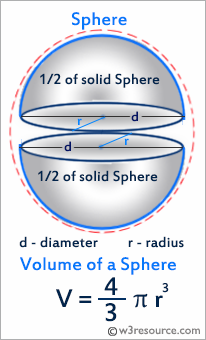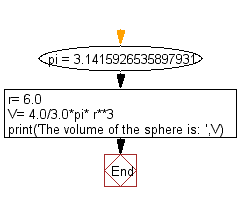﻿ Python: Get the volume of a sphere with radius 6 - w3resource# Python: Get the volume of a sphere with radius 6

## Python Basic: Exercise-15 with Solution

Write a Python program to get the volume of a sphere with radius 6.

Python: Volume of a Sphere

A sphere is a three-dimensional solid with no face, no edge, no base and no vertex. It is a round body with all points on its surface equidistant from the center. The volume of a sphere is measured in cubic units.

The volume of the sphere is : V = 4/3 × π × r3 = π × d3/6.Sample Solution :-

Python Code:

``````
pi = 3.1415926535897931
r= 6.0
V= 4.0/3.0*pi* r**3
print('The volume of the sphere is: ',V)
```
```

Sample Output:

```The volume of the sphere is:  904.7786842338603
```

Flowchart:## Visualize Python code execution:

The following tool visualize what the computer is doing step-by-step as it executes the said program:

Python Code Editor:

Have another way to solve this solution? Contribute your code (and comments) through Disqus.

What is the difficulty level of this exercise?

Test your Python skills with w3resource's quiz

﻿

## Python: Tips of the Day

Unpack variables from iterable:

```# One can unpack all iterables (tuples, list etc)
>>> a, b, c = 1, 2, 3
>>> a, b, c
(1, 2, 3)

>>> a, b, c = [1, 2, 3]
>>> a, b, c
(1, 2, 3)
```